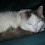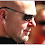## Sunday, April 28, 2019

### x3 + y3 + z3 = 33 has a solution in Z. And its big!

Consider the following problem:

Given k, a natural number, determine if there exists x,y,z INTEGERS such that x3+y3+z3=k.

It is not obvious that this problem is decidable (I think it is but have not been able to find an exact statement to that affect; however, if it was not solvable, I would know that, hence it is solvable. If you know a ref give it in the comments.)

If k≡ 4,5 mod 9 then mod arguments easily show there is no solution. Huisman showed that if k≤ 1000, k≡1,2,3,6,7,8 mod 9 and max(|x|,|y|,|z|) ≤ 1015 and k is NOT one of

33, 42, 114, 165, 390, 579, 627, 633, 732, 795, 906, 921, 975

then there was a solution. For those on the list it was unknown.

Recently Booker (not Cory Booker, the candidate for prez, but Andrew Booker who I assume is a math-computer science person and is not running for prez) showed that

x3 + y3 + z3 =33

DOES have a solution in INTEGERS. It is

x= 8,866,128,975,287,528

y=-8,778,405,442,862,239

z=-2,736,111,468,807,040

does that make us more likely or less likely to think that

x3 + y3 + z3 =42

has a solution? How about =114, etc, the others on the list?

Rather than say what I think is true (I have no idea) here is what I HOPE is true: that the resolution of these problems leads to some mathematics of interest.

1.you should know about #fermat therom which can be a genaralization of you want to solve. you should know that #andrew jhon wiles prof that it is not possible for all k>=3.

1.My post is about x^3 + y^3 + z^3 = 33, or other constants.
FLT is about x^3 + y^3 = z^3.

So-- did I say something incorrect in my post or are you saying that I should mention FLT since the equation looks similar?

2.What's the intuition behind your conjecture that the problem is decidable? I would have thought that the surprisingly large k=33 solution suggests undecidability.

3.Not sure I call this intuition, but here is what I think:
The proof that classes of Diophantine equations are undecidable seems to either need lots of variables or high degree. Since the equation featured in this blog post is only 3 vars of degree 3 it would seem hard to PROVE undecidability. AH- but the fact that the numbers are so large may well indicate that the TRUTH is undecidable.

1.But the degree of the equation can be reduced to 2 if we use the following identity. n^3 = T_n*T_n - T_(n-1)*T_(n-1), where T_n are triangular numbers. So now the machinery of quadratic diophantine equations can be used to tackle the question of undecidability ( hopefully).

4.(-80538738812075974)3 + 804357581458175153 + 126021232973356313 = 42

1.Wow, really? This is amazing! ;)

5.Its (-80538738812075974)^3 + 80435758145817115^3 + 12602123297335631^3 = 42

6.x^3+y^3+z^3=390
routrouve x y z ?

7.There is nothing impossible!

8.(8,866,128,975,287,528^3)+(-8,778,405,442,862,239)^3 +(-2,736,111,468,807,040)^3 = 0

9.